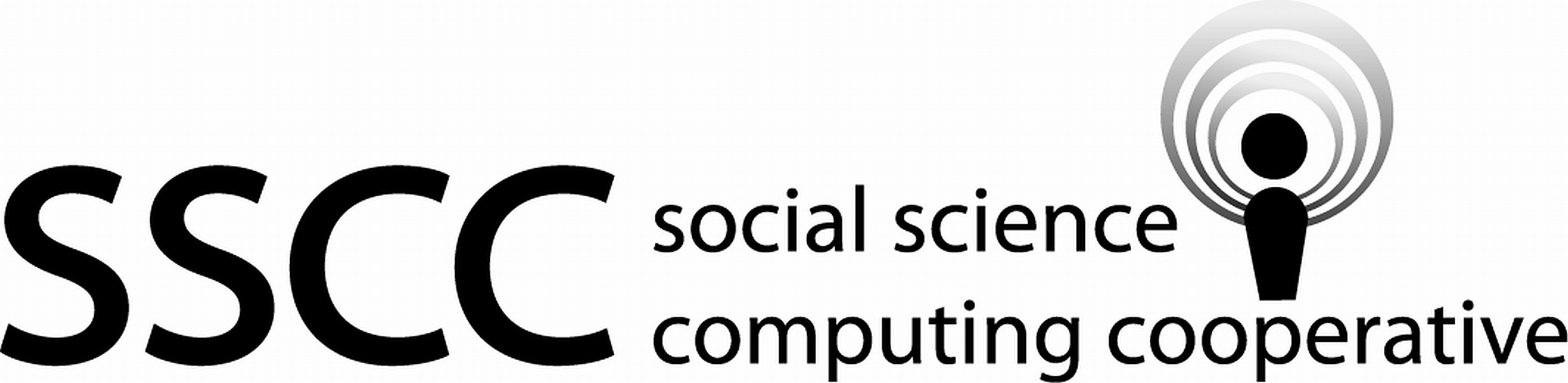Supporting Statistical Analysis for Research

## 3.2 Introduction

Data wrangling is the act of preparing data for further analysis. This definition requires a definition of what kind of data and what needs to be done to the data. We review these two questions before beginning the descriptions.

In this chapter data is structured data in tables, we refer to these as data frames. Date frames structure data in rows and columns This is not the only form data can take. Data can be structure as records. This is how data is structured in SQL. Record formatted data and data frames are similar in a number of ways. Their main differences are in how the data Is stored and modified. Data can also be unstructured. Text data is a common example of unstructured data. The position of a word within a text, paragraph, or sentences tells one little of how the word relates to the other words. This chapter only covers wrangling data frames.

What needs to be done varies from data frame to data frame and analysis to analysis. This chapter covers a set of wrangling tools that are applicable to many wrangling needs. It is by no means a complete set of wrangling tools. It is a quick introduction the structured approached to wrangling that is provided by the tidyverse.

Note, this chapter covers some of the same functions as the Introduction to the tidyverse. The focus in this chapter is using the tidyverse to wrangle data. Where as the focus of the Introduction to the tidyverse chapter is the functions.

Example

1. Loading the tidyverse package

library(tidyverse)